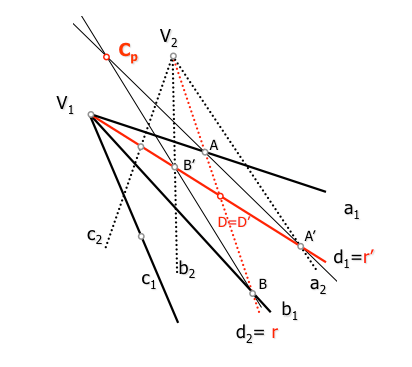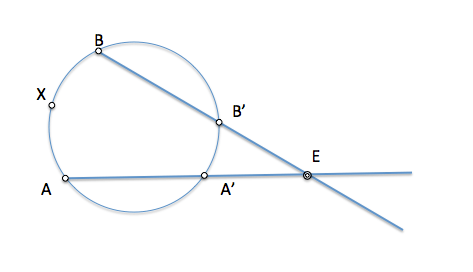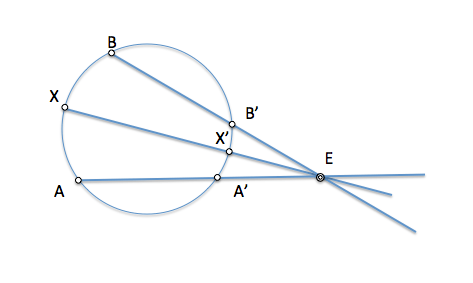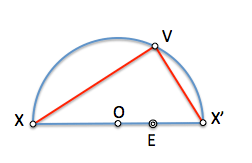# Geometría proyectiva : Center of involutionInvolutions in second-order series are of particular interest in the determination of the elements of a conical.

We have seen how to determine the axis of an involution and, based on the concept of Polar of a point with respect to two lines, possible Involutions which can be set from four points, with their respective axes of involution, obtaining the autopolar triangle Associate in which we find the harmonious relations of the full cuadrivertice.

In this article we will continue to enhance these elements, in particular in the autopolar triangle vertices that will determine what is known as “Center of involution”.

We will remember that two beams projective straight have a projective center It binds them. We can determine this point using the intersection of two loci (they will go through two perspectives series points result from section beams by homologous elements).

If we consider the points of intersection of pairs of associated lightning (a-b’ and ’-b) We will obtain the aforementioned lociProjective center of two projective bundles

If we project from any two points of a conical two superimposed series that are projective, the resulting beams are projective and will associate a projective Center.

In the figure we have projected from V1 and V2 points A,B,X …. and ’,B ’,X’ you are in involution. The pairs of associated lightning a-x’ and ’-x will determine a locus that is projective shaft of these bundles. This locus is the line a-a.’ that unites the two homologous points. Repeat this operation with another pair of points in involution we see that D3 will be the projective searched and each pair of homologous points in the regression will be on a line passing through this point, I will call “Center of involution”.If you get new points in any of the Involutions of axes e12, studied e23 and e31, We see that the pairs of homologous points will be aligned with the vertices of the triangle autopolar, D1, D2 and D3. In each involution pairs of homologous points will be on lines containing its axis of involution.This point will allow us to obtain the counterpart of a point on the regression with least laborious paths. We can for example use the Center and axis of involution in the same problem, highlighting how to operate with them, to determine the counterpart of a point X.

Is the involution of points a-a.’ and b-b’ which aims to determine the homologue of the point X.We will determine this point using the intersection of two loci in which must be.

• In the line that is formed by projecting X from the Centre of involution
• In the homologous spoke that we get to the project from a point of the Conic. Beam perspective with vertex in the homologous point of the projection will be axis perspective axis of involution.Even if we save a single line with respect to the use of the axis of involution, applied concepts will be us very useful in more complex problems as we will see later.

### Example: involution of points

Given involution is points a-a. ’, B-B’ on a circumference, determine counterpart of the point XWe determined the center of involution, to be found at the intersection of two loci: the straight lines containing each pair of homologous points.Counterpart of the point X will be in the circumference and the line containing X and the Centre of involution### Example: Involution of straight lines.

Given the involution of the straight a-a. ’, b-b’, determine the straight counterparts in involution which are perpendicular.

This exercise will be useful to later obtain a conical shafts from two pairs of conjugate diameters.We sectioned by a circle passing through the apex of the beam in involution, to determine two series of second order in involution.We can determine the elements of the involution, as the Center or axis as we have seen in studying these transformations. In this case you want to determine the Center and involution.We will remember that the concept of orthogonality of straight lines is associated to the of arc able 90 °, a semicircle.

If we take any point in a semicircle, point V, the straight lines determined by this point and ends x-x’ their diameter they are orthogonal.

VX and VX’ counterparts in an investment will be if the straight line x-x’ It contains the Center and involution.

Consequently X and X’ they must be in the diameter of the circle that contains the center of involution.Therefore, We will determine the solution to getting this diameter, simply from the center of the circumference and the point E. The solutions will be the straight lines x and x’